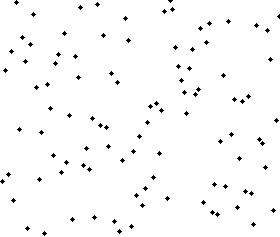01-28-2018A merge sort algorithm

HISTORY

The merge sort algorithm is an efficient divide and conquer sorting algorithm. It was invented by John von Neumann in 1945. John von Neumann (1903 - 1957) was a Hungarian-American mathematician, physicist and computer scientist. He is considered one of the greatest mathematicians of his time.John von Neumann

HOW IT WORKS

This algorithm is a divid and conquer type. This means that problem is divided into smaller subproblems for which a solution is very simple. There are two parts, one the divide part and then the merge part. The input array is divided into half and recursively called on each half. The base case is the empty array. Once the sub part is only one item long, the merging starts. Two sub sections are merged with the lower item taking the position before the higher item. All sub sections are merged until finally the last two halves are merged.

RUN TIME

What is the run time for this algorithm? The merge part of the algorithm takes O(n) time because the array needs to be traversed once, assigning the lower of the two items to the current array position. Now this merge step is done once for each of the divide steps. This means that the time complexity is O(n log n) because it takes log n for all the merge steps.

EXAMPLE DESCRIPTION

This is a python3 implementation of the merge sort algorithm. The divide step is done in the mergeSort function, while the merge step is done in the merge function.

Time Complexity:

• O(n log n)
```              ```
def mergeSort(arr, start, end):
if (start < end):
middle = start+((end-start)//2)
mergeSort(arr, start, middle)
mergeSort(arr, middle+1, end)
merge(arr, start, middle, end)

def merge(arr, start, middle, end):
leftHalf = arr[start:middle+1]
rightHalf = arr[middle+1:end+1]
i=0
j=0
k=start
while (i < len(leftHalf) and j < len(rightHalf)):
if (leftHalf[i] <= rightHalf[j]):
arr[k] = leftHalf[i]
i += 1
else:
arr[k] = rightHalf[j]
j += 1
k += 1
while (i < len(leftHalf)):
arr[k] = leftHalf[i]
i += 1
k += 1
while (j < len(rightHalf)):
arr[k] = rightHalf[j]
j += 1
k += 1

arr = [14, 7, 3, 12, 9, 11, 6, 2]
n = len(arr)
mergeSort(arr,0,n-1)
print(arr) # result equals [2, 3, 6, 7, 9, 11, 12, 14]

```
```